# 13 Electric Circuits Chapter Outline 1 Electric Circuits

• Slides: 1713 Electric CircuitsChapter Outline 1 Electric Circuits and Electric Current 2 Ohm’s Law and Resistance 3 Series and Parallel Circuits 4 Electric Energy and Power 5 Alternating currents and Household Current Everyday Phenomenon: The Hidden Switch in Your ToasterHow to Get the Bulb to Light?How to Get the Bulb to Light?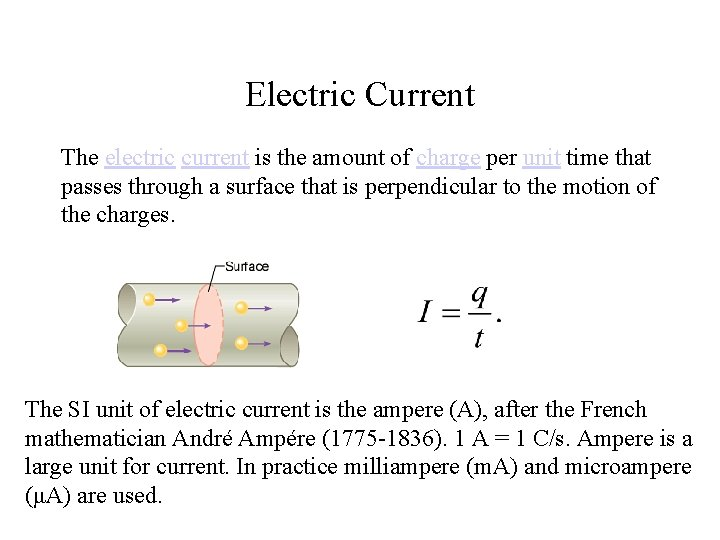Electric Current The electric current is the amount of charge per unit time that passes through a surface that is perpendicular to the motion of the charges. The SI unit of electric current is the ampere (A), after the French mathematician André Ampére (1775 -1836). 1 A = 1 C/s. Ampere is a large unit for current. In practice milliampere (m. A) and microampere (μA) are used.Direction of Current Flow Electric current is a flow of electrons. In a circuit, electrons actually flow through the metal wires. Conventional electric current is defined using the flow of positive charges. It is customary to use a conventional current I in the opposite direction to the electron flow.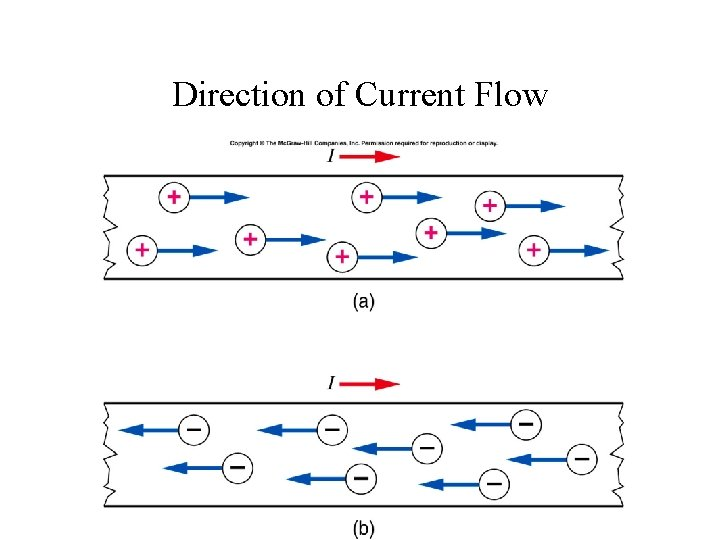Direction of Current FlowWhat Limits the Flow of Current?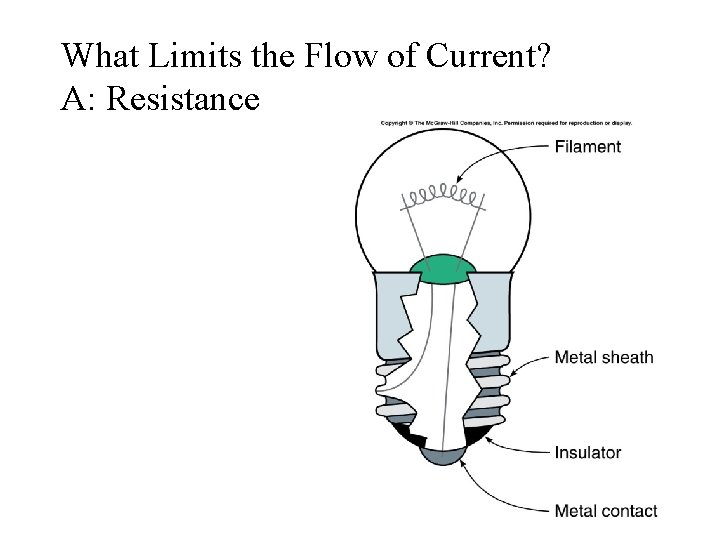What Limits the Flow of Current? A: Resistance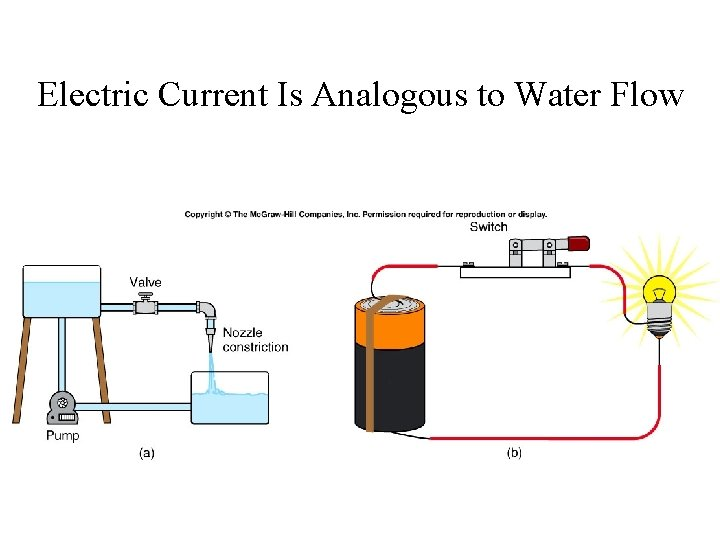Electric Current Is Analogous to Water FlowOhm’s Law Georg Simon Ohm (1787 -1854), a German physicist, discovered Ohm’s law in 1826. This is an experimental law, valid for both alternating current (ac) and direct current (dc) circuits. When you pass an electric current (I) through a resistance (R) there will be a potential difference or voltage (V) created across the resistance. Ohm’s law gives a relationship between the voltage (V), current (I), and resistance (R) as follows: V=IR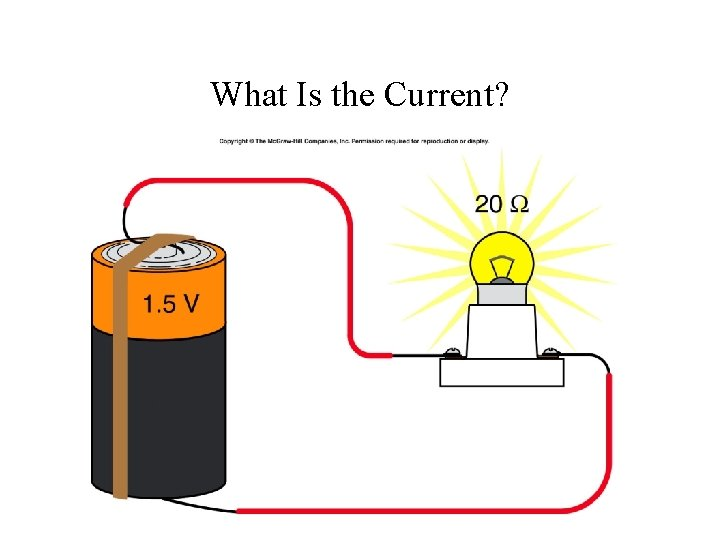What Is the Current?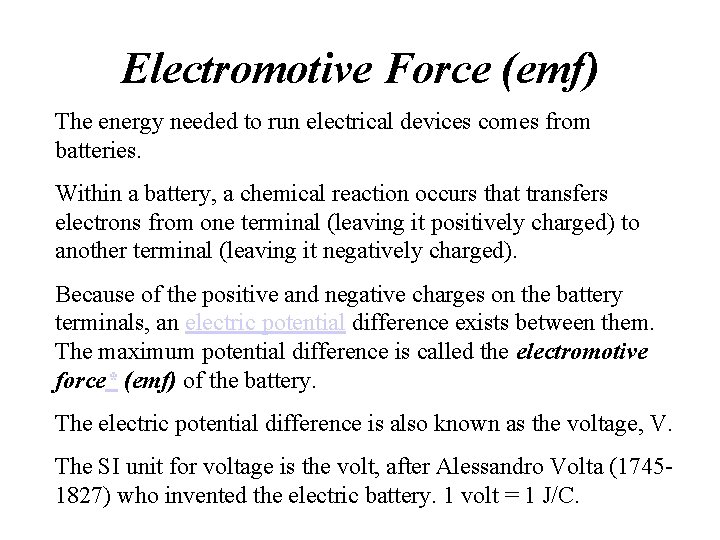Electromotive Force (emf) The energy needed to run electrical devices comes from batteries. Within a battery, a chemical reaction occurs that transfers electrons from one terminal (leaving it positively charged) to another terminal (leaving it negatively charged). Because of the positive and negative charges on the battery terminals, an electric potential difference exists between them. The maximum potential difference is called the electromotive force* (emf) of the battery. The electric potential difference is also known as the voltage, V. The SI unit for voltage is the volt, after Alessandro Volta (17451827) who invented the electric battery. 1 volt = 1 J/C.CircuitsSeries Circuit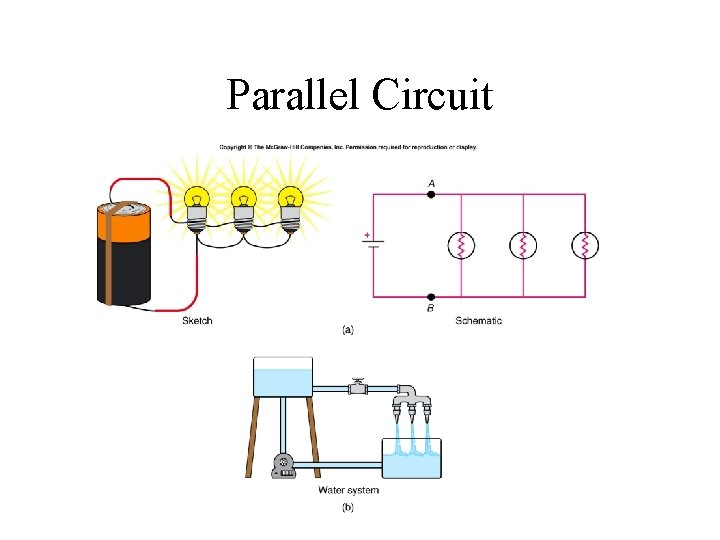Parallel Circuit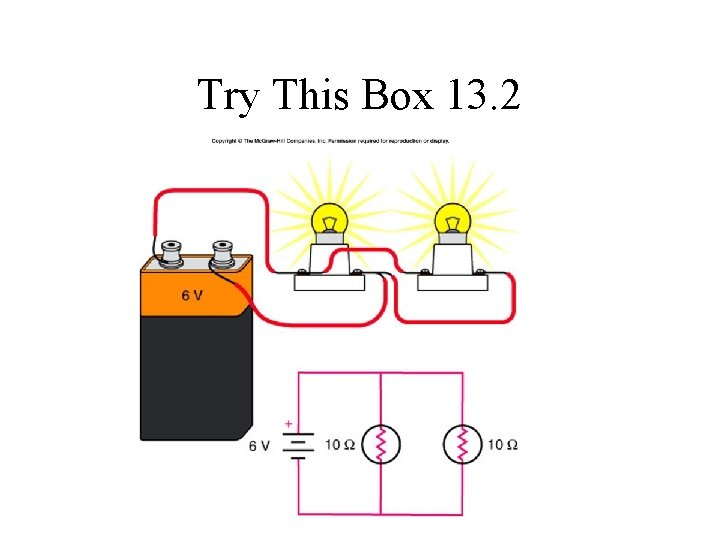Try This Box 13. 2